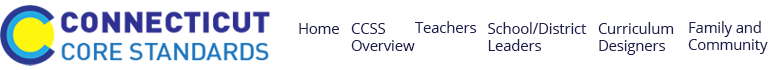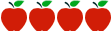### Grade 8: Identifying Similar Triangles

Rating:#### Common Core Standards

Content Standards

CCSS.Math.Content.8.G.A.5 Use informal arguments to establish facts about the angle sum and exterior angle of triangles, about the angles created when parallel lines are cut by a transversal, and the angle-angle criterion for similarity of triangles.

Standards for Mathematical Practice

MP1: Make sense of problems and persevere in solving them

MP2: Reason abstractly and quantitatively

MP3: Construct viable arguments and critique the reasoning of others

#### Description of Unit

This lesson titled "Identifying Similar Triangles" from map.mathshell.org is intended to teach students how to reason about geometry, particularly in regards to ideas and concepts related to similarity. In this lesson, students will use facts about the angle sum and exterior angles of triangles to calculate missing angles and apply angle theorems to parallel lines cut by a transversal. Students will also need to interpret geometrical diagrams using mathematical properties to identify similarity of triangles.

#### Cautions

Connecticut teachers should be aware that they would need to include supports for students working above/below grade level, those students who are identified as students with disabilities and for English language learners (ELLs). Teachers should make connections to other domains across the geometry standards that have been taught in previous grades, as well as grade 8. Also, no rubric is provided for sample solutions.

#### Rationale for Selection

This lesson attends to the rigor of the Common Core, requiring students to construct arguments in regards to similar triangles and discuss reasoning with others. The instructional support in the lesson plan is extensive, including sample student responses, and there are a multitude of resources presented to appropriately assess student progress. The lesson also provides supporting questions the teacher could ask students during the lesson to enhance student thinking.